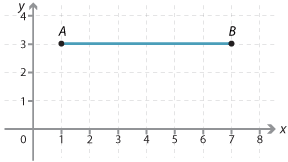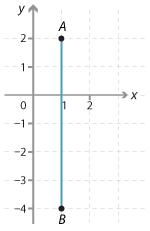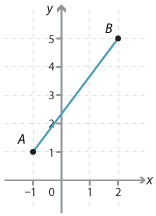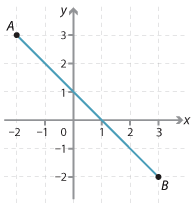#### Question 1

The distance between the following pairs of points is:

 a bA(1, 3) and B(7, 3) 5 6 7A(1, –4) and B(1, 2) 5 6 7

#### Question 2

The distance between the following pairs of points is:

 a bA(–1, 1) and B(2, 5) 3 4 5A(-2, 3) and B(3, -2) 5 $$2\sqrt5$$ $$2\sqrt10$$